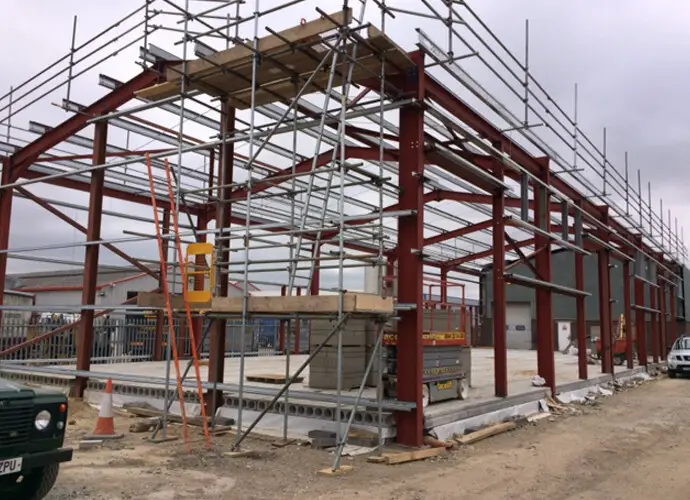# Buckling Amplification Factor for Portal Frames Sensitive to 2nd Order EffectsIn order to evaluate the sensitivity of a portal frame to 2nd order effects, the buckling amplification factor αcr has to be calculated. This calculation requires the deflections of the frame to be known under a given load combination. Check §5.2.1 of EN 1993-1-1.

In this post, elastic first order analysis is performed on a single bay portal frame using Staad.Pro in order to calculate the reactions under vertical loads at ULS (see figure below). The actions on the portal frames are as given below;

gk = 2.31 kN/m
qk = 3 kN/m

Design load = (1.35 gk) + (1.5 × qk)

Roof load = (1.35 × 2.31) + (1.5 × 3.0) = 3.1185 + 4.5 = 7.6185 kN/m

When analysed;

Vertical base reaction VEd = 118.263 kN
Horizontal base reaction HEd = 65.651 kN
Maximum Axial Load on rafter NR,Ed = 93.9 kN

Axial Compression in the rafter
According to clause 5.2.1(4), if the axial compression in the rafter is significant, then the αcr is not applicable.

The axial compression is significant if;

λ ̅  ≥ 0.3√((Afy)/NEd ) and this can be rearranged to show that the compression is significant if
NEd ≥ 0.09Ncr

NEd is the design axial load in the rafter
Lcr is the developed length of the rafter pair from column to column;
Lcr = 30/cos 15° = 31.058m
Ncr = (π2EI)/Lcr2 = (π2 × 210000 × 16000 × 104)/310582 = 343789.059 N = 344 kN

0.09Ncr = 0.09 × 344 = 30.94 kN
NEd = 93.9 kN > 30.94 kN, therefore axial load is significant.

When the axial force in the rafter is significant, a conservative measure of frame stability defined as αcr,est may be calculated. For frames with pitched rafters;

αcr,est = min(αcr,s,est ; αcr,r,est)

Where;
αcr,s,est is the estimate of αcr for the sway buckling mode
αcr,r,est is the estimate of αcr for the rafter snap-through buckling mode. This is only relevant when the frame has more than two bays or if the rafter is horizontal.

To calculate αcr, a notional horizontal force (NHF) is applied to the frame, and the horizontal deflection of the top of the column is determined under this load.

HNHF = VEd/200 = 118.263/200 = 0.591 kN

For the assessment of frame stability and for the assessment of deflections at SLS, the base may be modelled with a stiffness assumed to be a proportion of the column stiffness as follows;

• 10% when assessing frame stability (10% of the column stiffness may be modelled by using a spring stiffness equal to 0.4EIcolumn/Lcolumn)
• 20% when calculating deflections at SLS (20% of the column stiffness may be modelled by using a spring stiffness equal to 0.8EIcolumn/Lcolumn)

When the software cannot accommodate a rotational spring, the base fixity may be modelled by a dummy member of equivalent stiffness as shown below;

• For assessing frame stability, the second moment of area of the dummy member should be taken as Ixx = 0.1Ixx,column
• For calculating deflection at SLS, the second moment of area of the dummy member should be taken as Ixx = 0.2Ixx,column

In both cases, the length of the dummy member is 0.75Lcolumn and pinned at the far end.

On modelling the nominally pinned base using dummy members, the deflection below was obtained for the notional horizontal forces.

Therefore αcr = h / 200δNHF = 7000/(200 × 2.963) = 11.81
αcr,s,est = 0.8(1 – NEd/Ncr)
αcr = 0.8(1 – 93.9/344)11.81 = 6.869
αcr,s,est = 6.869 < 10

Therefore, second order effects are significant. Since αcr,s,est ≥ 3.0, the amplifier is given by;
[1/(1 – 1⁄αcr,est)] = [1/(1 – 1 ⁄ 6.869)] = 1.17

Note: If αcr,s,est is less than 3.0, second order analysis must be used. The simple amplification is not sufficiently accurate.

Therefore the modified partial factor of safety to account for second order effects are as follows;

γG = 1.17 × 1.35 = 1.5
γQ = 1.17 × 1.5 = 1.75

You can now use these modified partial factors to multiply the characteristic permanent and variable actions. The ultimate vertical action on the rafter (taking into account second order effects)is;

Roof load = (1.5 × 2.31) + (1.75 × 3.0) = 8.715 kN/m

Thank you for visiting Structville today and God bless you.

#### 1 COMMENT

1. • I have to admit that those faces look pretty grim. How anyone could go under the knife, knowing they may come out looking even worse, I don’t know